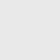# Binary to Decimal Converter

Easily convert the binary number to decimal number by using our converter TO-Tools.

## How to convert Binary to Decimal by manual calculation

There are two methods to convert a binary number to a decimal number system.

### Binary to Decimal Conversion Using Positional Notation Method

In any binary number, the rightmost digit is called the ‘Least Significant Bit’ (LSB) and the left-most digit is called the ‘Most Significant Bit’ (MSB). For a binary number with ‘n’ digits, the least significant bit has a weight of 20 and the most significant bit has a weight of 2n-1.

#### Let us take one simple example, Consider the binary number (10101)2

• Step 1: List out the powers of 2 for all the digits starting from the right-most position. The first power would be 20 and as we move on it will be 21, 22, 23, 24, 25,… In the given example, there are 6 digits, therefore, starting from the right-most digit, the weight of each position from the right is 20,21,22,23,24,25.
• Step 2: Now multiply each digit in the binary number starting from the right with its respective weight based on its position and make the formula and evaluate the product. As shown below.

(101010)=  (1⋅25) + (0⋅24) + (1⋅23) + (0⋅22) + (1⋅21) + (0⋅20)  =  (42)10

• Step 3: Now, We express the binary number as a decimal number:

(101010)2 = (42)10

### Binary to Decimal Conversion Using Doubling Method

Let us use the same example for converting the binary number (101010)2 to decimal.

• Step 1: Write the binary number and start from the left-most digit. Double the previous number and add the current digit. Since we are starting from the left-most digit and there is no previous digit to the left-most digit, we consider the double of the previous digit as 0. For example in (101010)2, the left-most digit is ‘1’. The double of the previous number is 0. Therefore, we get [(0 × 2) + 1] which is 1.
• Step 2: Continue the same process for the next digit also. The second digit from the left is 0. Now, double the previous digit and add it with the current digit. Therefore, we get, [(1 × 2) + 0], which is 2.
• Step 3: Continue the same step in sequence for all the digits. The sum that is achieved in the last step is the actual decimal value. As shown in the table. Therefore, the result of converting the binary number to a decimal using the doubling method is
(101010)2 = (42)10.

## Subscribe to our weekly newsletter

Subscribe
Notify ofInline Feedbacks

## Browse## Browse